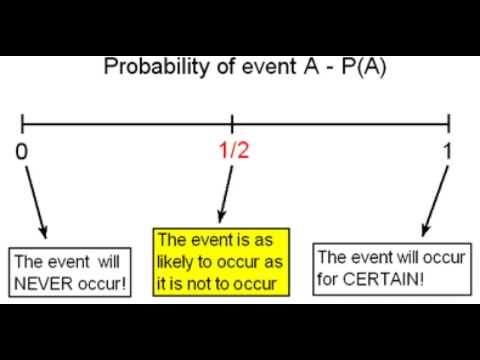# 6.9: Another Look at Probability (1 of 2)

$$\newcommand{\vecs}{\overset { \rightharpoonup} {\mathbf{#1}} }$$ $$\newcommand{\vecd}{\overset{-\!-\!\rightharpoonup}{\vphantom{a}\smash {#1}}}$$$$\newcommand{\id}{\mathrm{id}}$$ $$\newcommand{\Span}{\mathrm{span}}$$ $$\newcommand{\kernel}{\mathrm{null}\,}$$ $$\newcommand{\range}{\mathrm{range}\,}$$ $$\newcommand{\RealPart}{\mathrm{Re}}$$ $$\newcommand{\ImaginaryPart}{\mathrm{Im}}$$ $$\newcommand{\Argument}{\mathrm{Arg}}$$ $$\newcommand{\norm}{\| #1 \|}$$ $$\newcommand{\inner}{\langle #1, #2 \rangle}$$ $$\newcommand{\Span}{\mathrm{span}}$$ $$\newcommand{\id}{\mathrm{id}}$$ $$\newcommand{\Span}{\mathrm{span}}$$ $$\newcommand{\kernel}{\mathrm{null}\,}$$ $$\newcommand{\range}{\mathrm{range}\,}$$ $$\newcommand{\RealPart}{\mathrm{Re}}$$ $$\newcommand{\ImaginaryPart}{\mathrm{Im}}$$ $$\newcommand{\Argument}{\mathrm{Arg}}$$ $$\newcommand{\norm}{\| #1 \|}$$ $$\newcommand{\inner}{\langle #1, #2 \rangle}$$ $$\newcommand{\Span}{\mathrm{span}}$$$$\newcommand{\AA}{\unicode[.8,0]{x212B}}$$

Learning Objectives

• Interpret (in context) a probability as a long-run relative frequency of an event.

In the module Relationships in Categorical Data with Intro to Probability, we used the word probability to mean “likelihood” or “chance.” We used data to make statements about

• the likelihood that a randomly selected student from a specific college is a Health Science major.
• the risk associated with not wearing a seat belt.
• the chance of a positive drug test for someone who does not use drugs when the test is 94% accurate.

For each of these probability statements, we used a notation P(A) where A is the description of an event. We used the following notation to represent probability statements like the preceding ones:

• P(Health Science)
• P(fatal accident given that the person was not wearing a seatbelt) = P(fatal accident | not wearing a seatbelt)
• P(a person is not a drug user given that the person had a positive test result) = P(not a drug user | positive test result)

In each case, the probability was a number between 0 and 1. What does this number tell us about the likelihood of an event occurring?A YouTube element has been excluded from this version of the text. You can view it online here: pb.libretexts.org/cis/?p=242

In summary, the probability that an event will occur is a number between (and including) 0 and 1. We write this idea in mathematical notation as 0 ≤ P(A) ≤ 1.

## A Closer Look at How We Calculate Probabilities

You may have had some experience with probability using coins, cards, and dice.

• What is the probability that when you flip a coin you get heads?
• What is the probability that when you roll the dice you get doubles?

We can answer these types of probability questions without collecting data. In situations where the outcomes are equally likely, we can use mathematics to calculate the probability instead of collecting data. For example, what is the probability of getting heads when you toss a coin? There are two equally likely outcomes: heads or tails. So$P(\mathrm{heads})=\frac{1}{2}$. This is the theoretical probability of getting a head when you toss a coin. We determine the number of ways an event can occur and divide by the total number of possible outcomes. No experiments or data collection is necessary.

What is the probability that a community college student is female? Like tossing a coin, this event also has two outcomes: female or male. But is$P(\mathrm{female})=\frac{1}{2}$ ? To estimate this probability, we have to collect data. We can use the data from the West Coast college that we saw in Relationships in Categorical Data with Intro to Probability and estimate that$P(\mathrm{female})=\frac{\mathrm{6,198}}{\mathrm{12,000}}=0.5165$ . Of course, this estimate assumes this college is a representative sample of community colleges. Data from 2010 enrollments at Los Medanos College in California give a different estimate:$P(\mathrm{female})=\frac{\mathrm{5,581}}{\mathrm{9,966}}=0.56$ . Neither estimate is equal to 0.5 because there appear to be more women than men attending community college. These are examples of empirical probabilities.

Empirical probability of an event is an estimate, using data, of the likelihood that the event will happen. We can view the probabilities we calculated in Relationships in Categorical Data with Intro to Probability as empirical probabilities.